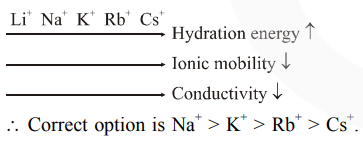# The correct order of conductivity of ions in water is :

Question:

The correct order of conductivity of ions in water is :

1. $\mathrm{Na}^{+}>\mathrm{K}^{+}>\mathrm{Rb}^{+}>\mathrm{Cs}^{+}$

2. $\mathrm{Cs}^{+}>\mathrm{Rb}^{+}>\mathrm{K}^{+}>\mathrm{Na}^{+}$

3. $\mathrm{K}^{+}>\mathrm{Na}^{+}>\mathrm{Cs}^{+}>\mathrm{Rb}^{+}$

4. $\mathrm{Rb}^{+}>\mathrm{Na}^{+}>\mathrm{K}^{+}>\mathrm{Li}^{+}$

Correct Option: , 2

Solution:As the size of gaseous ion decreases, it get more hydrated in water and hence, the size of aqueous ion increases. When this bulky ion move in solution, it experience greater resistance and hence lower conductivity.

Size of gasesous ion : $\mathrm{Cs}^{+}>\mathrm{Rb}^{+}>\mathrm{K}^{+}>\mathrm{Na}^{+}$

Size of aqueous ion : $\mathrm{Cs}^{+}<\mathrm{Rb}^{+}<\mathrm{K}^{+}<\mathrm{Na}^{+}$

Conductivity : $\mathrm{Cs}^{+}>\mathrm{Rb}^{+}>\mathrm{K}^{+}>\mathrm{Na}^{+}$